Latest Banking jobs   »   Quantitative Aptitude Quiz For IBPS Clerk/PO...

# Quantitative Aptitude Quiz For IBPS Clerk/PO Prelims 2022- 12th September

Q1. Circumference of a circle is 44 cm and radius of a cylinder is 500/7% of the diameter of the circle. If height of the cylinder is 14 cm, then find the volume (in cm³) of the cylinder?
(a) 4400 cm³
(b) 4290 cm³
(c) 4620 cm³
(d) 4200 cm³
(e) 4070 cm³

Q2. P & Q stared a business by investing Rs.5400 and Rs.4500 respectively. After four months P withdrew Rs.400 from his initial investment. If after one year the profit share of P is Rs.9240, then find the total profit?
(a) Rs.17480
(b) Rs.17340
(c) Rs.17240
(d) Rs.17640
(e) Rs.17260

Q3. The present age of Y is 28 years and four years hence the ratio of age of X to Y will be 7: 8. If present age of Z is 25% more than the present age of X, then find the age of Z six years ago?
(a) 26 years
(b) 22 years
(c) 20 years
(d) 24 years
(e) 28 years

Q4. Pipe P & Q together can fill a tank in 20 minutes, while pipe P, Q and R together can fill the same tank in 25 minutes. If Pipe Q & R together can fill the tank in 50 minutes, then in how many minutes pipe P alone can fill the same tank completely?
(a) 75 min
(b) 30 min
(c) 40 min
(d) 60 min
(e) 50 min

Q5. A train crosses a man running in opposite direction in 12 seconds. If speed of man and speed of train is 24 km/hr & 84 km/hr respectively, then find the length of train (in meters)?
(a) 324
(b) 360
(c) 344
(d) 380
(e) 310

Q6. The difference between marked price and selling price of an article is Rs.84 and the marked price of the article is 40% more than its cost price. If shopkeeper allowed 30% discount, then find the cost price of the article.
(a) Rs.250
(b) Rs.400
(c) Rs.150
(d) Rs.200
(e) Rs.450

Q7. 180 liters of mixture of milk and water is in the ratio of 7:5 respectively. If 15-liter milk and Y liters of water added in the mixture, then the ratio of milk to water becomes 6:5 respectively. Find the value of Y.
(a) 20
(b) 5
(c) 15
(d) 10
(e) 25

Q8. P is 25% more efficient than Q and R is 40% less efficient than P. If P and R together can complete a work in 12 days, then find the time taken by P, Q & R together to complete the same work.
(a) 12 days
(b) 6 days
(c) 9 days
(d) 8 days
(e) 15 days

Q9. X, Y and Z started a business by investing of Rs.2400, Rs.3000 and Rs.2600 respectively. After four months, X and Y withdrew his investment by 25% and 20% respectively. If at the end of the years Z received profit of Rs.1950, then find the profit share of X.
(a) Rs.1250
(b) Rs.1500
(c) Rs.1850
(d) Rs.1900
(e) Rs.1000

Q10. Ratio of present ages of A to B is 5: 4 and present age of C is 40% more than that of A. If the average of present ages of A, B & C is 16 years, then find the age of B six years ago.
(a) 6 years
(b) 15 years
(c) 8 years
(d) 12 years
(e) 4 years

Q11. The ratio of cost price to marked price of a table is 2 : 3 and shopkeeper allows two successive discounts of 100/9% and 10% on the marked price of the table. If the difference between profit earned and discount given is Rs. 200, then find the marked price of the table?
(a) Rs. 2100
(b) Rs. 3000
(c) Rs. 2400
(d) Rs. 3600
(e) Rs. 3300

Q12. The downstream speed of a boat is 5 km/hr more than its upstream speed and the ratio of the speed of the boat in still water to the speed of the stream is 19: 5. Find the total time taken by boat to travel 42 km downstream and 31.5 km upstream?
(a) 7 ½ hr
(b) 8 hr
(c) 9 hr
(d) 9 ½ hr
(e) 10 hr

Q13. A container contains a mixture of milk and water in which water is 40% less than milk. If 16 liter of the mixture is taken out and replaced with water, then the quantity of milk and water in final mixture becomes equal. Find the initial quantity of mixture in the container?
(a) 80 L
(b) 60 L
(c) 70 L
(d) 48 L
(e) 96 L

Q14. P and Q invested a total of Rs.32000 in a business. Period of investment of P & Q is 10 months and 8 months respectively. At the end of a year, P and Q received profit in the ratio 3 : 4 respectively. Find amount invested by P?
(a) Rs 20000
(b) Rs 18000
(c) Rs 14000
(d) Rs 12000
(e) Rs 16000

Q15. If diameter of the circle is equal to the side of the square having perimeter 112 cm, then find the difference between area of the square and that of the circle?
(a) 180 cm²
(b) 178 cm²
(c) 168 cm²
(d) 154 cm²
(e) 152 cm²

Solutions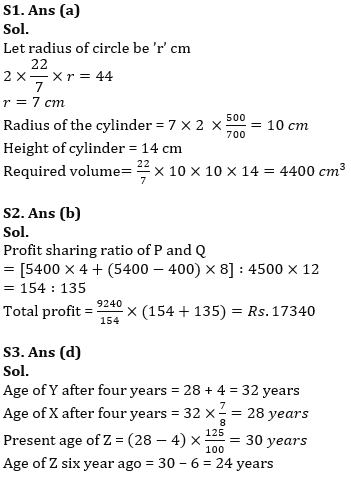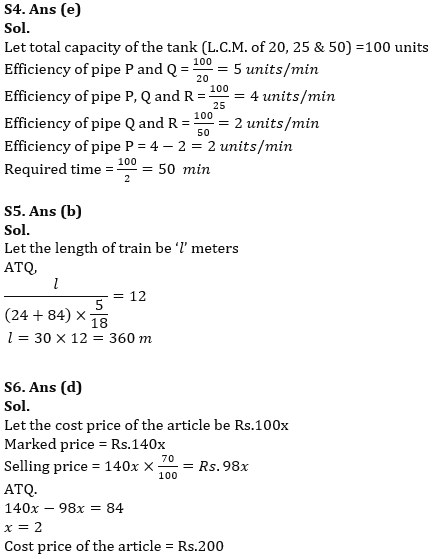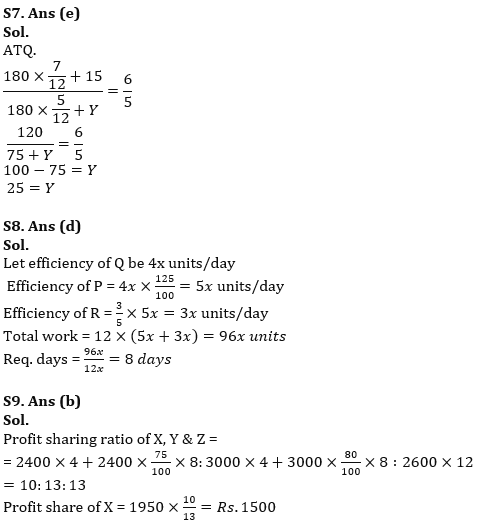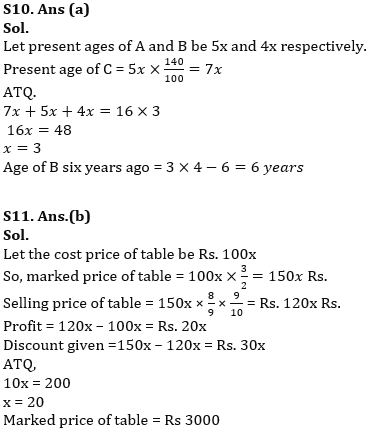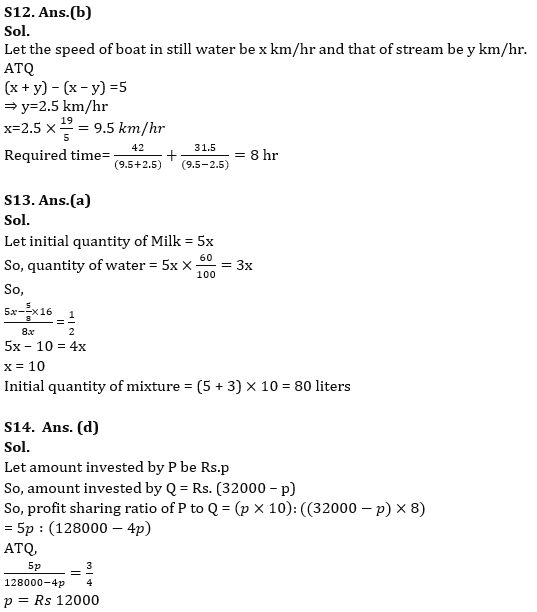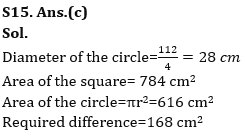#### Congratulations!Union Budget 2023-24: Free PDF